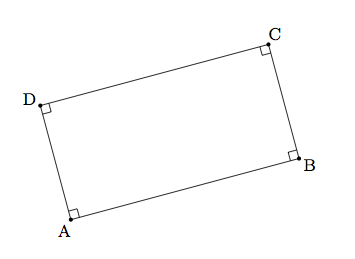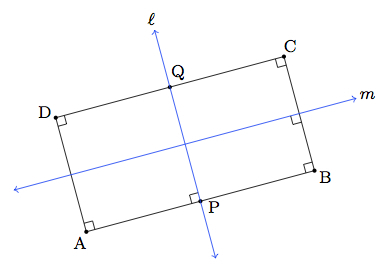# Symmetries of rectangles

Alignments to Content Standards: G-CO.A.3

Jennifer draws the rectangle $ABCD$ below:1. Find all rotations and reflections that carry rectangle $ABCD$ onto itself.
2. Lisa draws a different rectangle and she finds a larger number of symmetries (than Jennifer) for her rectangle. What can you conclude about Lisa's rectangle? Explain.

## IM Commentary

This task examines the rigid motions which map a rectangle onto itself. The emphasis here is on careful reasoning using the definitions of reflections and rotations. Students can use their geometric intuition (or experimentation with physical manipulatives) to find the four symmetries $ABCD$ (including the identity) and the main work in this task will be using the definitions of rotations and reflections to justify this intuition. The teacher may wish to prompt students to explain why their list of rigid motions is complete.

This task provides a good opportunity for students to engage in MP3, Construct Viable Arguments and Critique the Reasoning of Others. Putting together complete arguments explaining how the different rigid motions carry $ABCD$ to itself requires care and patience. Students can also use MP7, Look for and Make Use of Structure, since one way to create new rigid motions preserving $ABCD$ is to compose rigid motions already shown to preserve the rectangle: this is done in the solution where the two reflections are composed to yield the 180 degree rotation preserving $ABCD$.

## Solution

1. We first look for the reflections that map $ABCD$ onto itself. Since $ABCD$ is a rectangle, the line through the midpoints $P$ and $Q$ of the respective sides $\overline{AB}$ and $\overline{CD}$ is perpendicular to both $\overline{AD}$ and $\overline{BC}$. Since $A$ and $B$ are equidistant from $P$, reflection across $\ell$ interchanges $A$ and $B$, and similarly for $C$ and $D$. Thus the reflection also interchanges the rectangle edges $\overline{AD}$ and $\overline{BC}$, and leaves fixed the edges $\overline{AB}$ and $\overline{CD}$, so preserves the entire rectangle.We can apply the same argument to the perpendicular bisector $m$ of $\overline{BC}$, pictured below:In addition to the reflections $r_m$ and $r_\ell$ we can compose them: the rigid motion $r_\ell \circ r_m$ interchanges $A$ and $C$ and interchanges $B$ and $D$. This is a rotation by 180 degrees around the intersection of $\ell$ and $m$, the center of the rectangle.

We also need to explain why there are no other rigid motions, other than the identity, which preserve $ABCD$. A rigid motion of the plane which takes $ABCD$ to itself must interchange the vertices $A,B,C,$ and $D$ in some way. If, for example, $A$ maps to $A$ then $D$ must map to $D$ (as it is the only vertex whose distance from $A$ is $|AD|$) and similarly $B$ must go to $B$ and $C$ goes to $C$: this is the identity symmetry. A similar argument shows that there is only one way to send $A$ to $D$ (reflection about $\ell$), one way to send $A$ to $B$ (reflection about $m$), and one way to send $A$ to $C$ (the 180 rotation discussed above).

2. The argument to find the rigid motions carrying Jennifer's rectangle to itself applies to any rectangle, but the argument that these were the only four applied specifically to Jennifer's rectangle. Indeed, looking at the last paragraph of the solution to (a), when we argued that the only symmetry of $ABCD$ carrying $A$ to $A$ is the identity, we used the fact that $|AD| \neq |AB|$. If $|AD| = |AB|$, then $ABCD$ is a square. In this case, reflection over the diagonal $\overleftrightarrow{AC}$ is a symmetry of the square which is not a symmetry of the rectangle considered in part (a). We conclude that Lisa's rectangle must be a square.